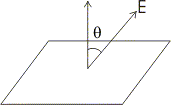# Electric flux

## 9. Electric Flux

• Consider a plane surface of area ΔS in a uniform electric field E in the space.

• Draw a positive normal to the surface and θ be the angle between electric field E and the normal to the plane.• Electric flux of the electric field through the choosen surface is then
Δφ = E ΔS cosθ

• Corresponding to area ΔS we can define an area vector ΔS of magnitude ΔS along the positive normal. With this definition one can write electric flux as
Δφ = E . ΔS

• direction of area vector is always along normal to the surface being choosen.

• Thus electric flux is a measure of lines of forces passing through the surface held in the electric field.

Special Cases
• If E is perpendicular to the surface i. e., parallel to the area vector then θ = 0 and
Δφ = E ΔS cos0

• If θ = π i. e., electric field vector is in the direction opposite to area vector then
Δφ = - E ΔS

• If electric field and area vector are perpendicular to each other then θ = π/2 and Δφ = 0

• Flux is an scaler quantity and it can be added using rules of scaler addition.

• For calculating total flux through any given surface , divide the surface into small area elements. Calculate the flux at each area element and add them up.

• Thus total flux φ through a surface S is
φ ≅ ΣE.ΔS

• This quantity is mathematically exact only when you take the limit ΔS→0 and the sum in equation 3 is written as integral
φ = ∫ΣE.dS